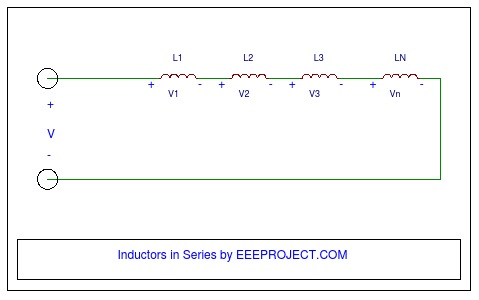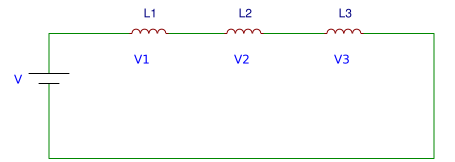# Inductors in Series [Explained] in Detail

Before starting off, we must know that what do we mean by an Inductor  in series. When the negative of first element is connected to positive of second and negative of second to positive of third and so on, then the connected elements are said to be in series. Also, the current flowing through the elements connected in series are always same.

Inductors can be connected in series or parallel or they can even be connected in the combination of both parallel and series. Here, in this article we will focus on inductors in series and later will learn about Inductors in parallel.### Equivalent Inductance of inductors in series

Let us see practically, what could be the equivalent inductance when number of inductors are connected in series. As shown in the circuit below, suppose we have three inductors connected in series across the dc battery having voltage V.Inductors in series

Take the individual inductances as L1, L2 & L3 having voltages V1, V2 & V3 respectively. Now the total voltage of the inductors added together must be equal to the voltage of the source battery,

i.e., V = V1 + V2 + V3                                   …..(1)

Also, we know that the voltage across an Inductor is given as:

VL = L (diL/dt)

Similarly,

V1 = L1 (di/dt),        V2 = L2 (di/dt),       V3 = L3 (di/dt)

putting the above values in eqn (1), we get

V  = L1 (di/dt) +  L2 (di/dt) + L3 (di/dt)       [using  “i” because current is same]

= (L1 + L2 + L3) (di/dt)

= Leqivalent (di/dt)

Therefore, we get Lequivalent = L1 + L2 + L3Equivalent circuit

The above calculation can now be generalized. The equivalent inductance of “n” number of inductors conneted in series having inductances as L1, L2, L3,….Ln can be replaced with an equivalent inductor having inductance as:

Lequi = (L1 + L2 + L3 + ….. Ln)

Also watch FM transmitter circuit

### Applications of inductors in series

• In electrical transmission systems for reducing the voltage that occurs through lightning strikes.
• For processing the signals in analog circuits.
• Used in transformers.

### Do U Know!

Capacity of inductors in series depends on the factors mentioned below:

1. The core around which the coil is wrapped.
2. Length of the coil: It is said that the smaller the coil, the more will be the inductance, as the coil will be narrower.
3. Cross-sectional area of coil: It is said that the inductance will be more if the area of cross-section of coil will be more.
4. Number of coils: More will be inductance with more number of coils.

You may also like to see the Types of Capacitors

FREE Electronic Projects List
Enter your email below and we will send it right after you hit the button below!
We respect your privacy. Your information is safe and will never be shared.•
•
•
•
•

## Join the discussion

1.Arshi Mehta

How can i calculate the resultant power stored in the inductors connected in series ?

•Ajay Dheeraj

Well you can use the formula as E=(1/2)L(I)^2, where L is the equivalent inductance and I will be the supply current.

Hey Wait ! Don't Go !!
You may like to have this amazing list of electronic projects
which we share with only few.